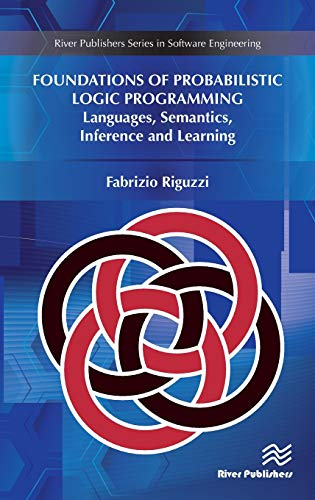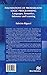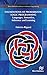Saturday , January 16th 2021

# Foundations of Probabilistic Logic Programming: Languages, Semantics, Inference and Learning (River Publishers Series in Software Engineering)#### Product Features:

Probabilistic Logic Programming extends Logic Programming by enabling the representation of uncertain information by means of probability theory. Probabilistic Logic Programming is at the intersection of two wider research fields: the integration of logic and probability and Probabilistic Programming. Logic enables the representation of complex relations among entities while…
Price as on: 2019-12-29 14:53:39

#### Product Description

Probabilistic Logic Programming extends Logic Programming by enabling the representation of uncertain information by means of probability theory. Probabilistic Logic Programming is at the intersection of two wider research fields: the integration of logic and probability and Probabilistic Programming.

Logic enables the representation of complex relations among entities while probability theory is useful for model uncertainty over attributes and relations. Combining the two is a very active field of study. Probabilistic Programming extends programming languages with probabilistic primitives that can be used to write complex probabilistic models. Algorithms for the inference and learning tasks are then provided automatically by the system.

Probabilistic Logic Programming is at the same time a logic language, with its knowledge representation capabilities, and a Turing complete language, with its computation capabilities, thus providing the best of both worlds.

Since its birth, the field of Probabilistic Logic Programming has seen a steady increase of activity, with many proposals for languages and algorithms for inference and learning. Foundations of Probabilistic Logic Programming aims at providing an overview of the field with a special emphasis on languages under the Distribution Semantics, one of the most influential approaches. The book presents the main ideas for semantics, inference, and learning and highlights connections between the methods.

Many examples in the book include a link to a page of the web application http://cplint.eu where the code can be run online.

## C++ Crash Course: A Fast-Paced

A fast-paced, thorough introduction to modern C++ written for experienced programmers. After reading C++ Crash Course, you'll be proficient in…

## The Language of SQL

The Language of SQL, Second Edition Many SQL texts attempt to serve as an encyclopedic reference on SQL…

## Python Programming for Beginners: Basic Language from

Have you been interested in getting started with coding, but you are worried that it will be too hard…